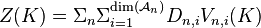# Notes for AKT-091020/0:22:01

Let$Z(K)=\Sigma_n \Sigma_{i=1}^{\dim(\mathcal{A}_n)} D_{n,i}V_{n,i}(K)$